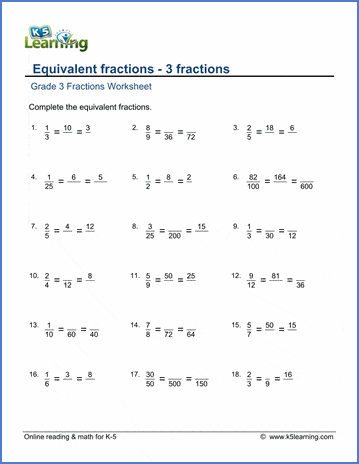# 3 Equivalent fractions

## Worksheets: Equivalent fractions (3 fractions per question)

Below are six versions of our grade 3 math worksheet on equivalent fractions; each question shows 3 equivalent fractions and the student must fill in the missing numerator or denominator .  These worksheets are pdf files.## More fractions worksheets

Explore all of our fractions worksheets, from dividing shapes into "equal parts" to multiplying and dividing improper fractions and mixed numbers.

## What is K5?

K5 Learning offers reading and math worksheets, workbooks and an online reading and math program for kids in kindergarten to grade 5.  We help your children build good study habits and excel in school.